Thursday, June 4, 2020
Home > Latest Announcement > NSEJS Maths Previous Year Questions Bank With Solutions

# NSEJS Maths Previous Year Questions Bank With SolutionsHi students, welcome to AmansMathsBlogs (AMB). On this article, you will get the collection of NSEJS Maths Previous Year Questions Bank With Solutions.

That means these are the questions collection with solutions asked in the NSEJS (National Standard Examination in Junior Science) exam.

You can download it as a PDF file. This PDF file contains the maths questions along with answer keys and solutions.

These questions are solved by me, AMAN RAJ, I am maths faculty of IIT Maths Foundation course and founder of this website AmansMathsBlogs (AMB).

NSEJS Maths Previous Year Questions Bank With Solutions: Ques No 03

If p + q + r = 2, p2 + q2 + r2 = 30 and pqr = 10, then the value of (1 – p)(1 – q)(1 – r) will be

[NSEJS 2017-18]

Options:

A. -18

B. -24

C. -27

D. -35

NSEJS Maths Previous Year Questions Bank With Solutions: Ques No 05

If ABCD is a cyclic quadrilateral, AB = 204, BC = 104, CD = 195, DA = 85 and BD = 221, then find AC.

[NSEJS 2017-18]

Options:

A. 240

B. 225

C. 220

D. 210

NSEJS Maths Previous Year Questions Bank With Solutions: Ques No 08

In triangle ABC, segment AD, segment BE and segment CF are altitude. If AB×AC = 172.8 cm2 and BE×CF = 108.3 cm2, then find AD×BC.

[NSEJS 2017-18]

Options:

A. 136.8 cm2

B. 132.4 cm2

C. 129.2 cm2

D. 128.6 cm2

NSEJS Maths Previous Year Questions Bank With Solutions: Ques No 11

If (x + 1/x) = 5, then find (x3 + 1/x3) – 5(x2 + 1/x2) + (x + 1/x)

[NSEJS 2017-18]

Options:

A. 0

B. 5

C. -5

D. 10

NSEJS Maths Previous Year Questions Bank With Solutions: Ques No 13

On seventy first Independence Day, there was Tuesday. After how many years there will be Tuesday on Independence Day?

[NSEJS 2017-18]

Options:

A. 4 Years

B. 5 Years

C. 6 Years

D. 7 Years

NSEJS Maths Previous Year Questions Bank With Solutions: Ques No 16

The sum of two numbers is 13 and the sum of their cubes is 1066. Find the product of those two numbers.

[NSEJS 2017-18]

Options:

A. 26

B. 27

C. 28

D. 29

NSEJS Maths Previous Year Questions Bank With Solutions: Ques No 18

A train is running at a speed of 54 km/hr. It is not stopping at a certain station. It crosses the person showing green flag in 20 seconds and crosses the platform in 36 seconds. What is the length of the train?

[NSEJS 2017-18]

Options:

A. 240 m

B. 300 m

C. 320 m

D. 360 m

NSEJS Maths Previous Year Questions Bank With Solutions: Ques No 23

The number of square formed by 5 vertical and 4 horizontal lines (all are equispaced) is

[NSEJS 2016-17]

Options:

A. 60

B. 20

C. 40

D. 46

NSEJS Maths Previous Year Questions Bank With Solutions: Ques No 26

In a n-sided regular polygon, the radius of the circumcircle is equal in length to the shortest diagonal. The number of values of n < 60 for which this can happen is

[NSEJS 2016-17]

Options:

A. 0

B. 1

C. 10

D. 2

NSEJS Maths Previous Year Questions Bank With Solutions: Ques No 31

A triangle has perimeter 316 and its sides are of integer length. The maximum possible area for such a triangle is achieved for

[NSEJS 2016-17]

Options:

A. Single triangle

B. Two triangle

C. Three triangle

D. More Than Three triangle

NSEJS Maths Previous Year Questions Bank With Solutions: Ques No 33

The number 38(310 + 65) + 23(212 + 67) is

[NSEJS 2016-17]

Options:

A. A perfect square and a perfect cube

B. Neither a perfect square nor a perfect cube

C. A perfect cube but not a perfect square

D. A perfect square but not a perfect cube

NSEJS Maths Previous Year Questions Bank With Solutions: Ques No 38

Two friends A and B watched a car from the top of their buildings. Angle of depression for A was 10° more than angle of depression for B, then

[NSEJS 2016-17]

Options:

A. A’s apartment is taller than B’s apartment

B. B’s apartment is taller than A’s apartment

C. A’s apartment and B’s apartment have same height

D. We cannot compare the heights of the two apartments.

NSEJS Maths Previous Year Questions Bank With Solutions: Ques No 45

The adjacent sides of a parallelogram are 30 cm and 20 cm. The length of one of the diagonals is 40 cm. What is length of the other diagonal?

[NSEJS 2015-16]

Options:

A. 60 cm

B. 10Root10 cm

C. 20Root5 cm

D. 8Root30 cm

NSEJS Maths Previous Year Questions Bank With Solutions: Ques No 50

8888888N8888888 this fifteen-digit number is divisible by 22. Find the eighth digit in the number

[NSEJS 2015-16]

Options:

A. 1

B. 3

C. 5

D. 8

NSEJS Maths Previous Year Questions Bank With Solutions: Ques No 51

In the adjoining figure, segment AD, Be and CF are the altitudes of triangle ABC. Find AD × BC, if AB×AC = 409.6 and BE×CF = 202.5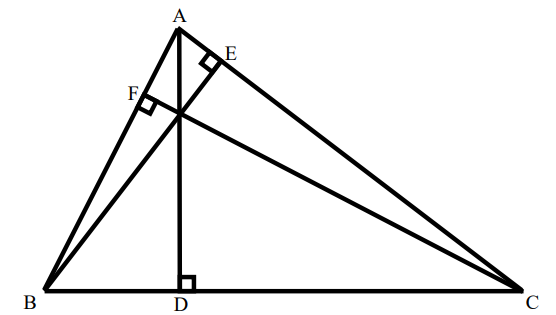[NSEJS 2015-16]

Options:

A. 225

B. 256

C. 288

D. 312

NSEJS Maths Previous Year Questions Bank With Solutions: Ques No 55

The sum of first four terms of an AP is 56. The sum of last four terms of same AP is 112. The first term of the AP is 11. Find the number of terms in that AP.

[NSEJS 2015-16]

Options:

A. 7

B. 8

C. 11

D. 13

NSEJS Maths Previous Year Questions Bank With Solutions: Ques No 60

Two parallel chords 96 cm and 28 cm long are on the opposite sides of the center of the circle with radius 50 cm. Find the area of the quadrilateral whose vertices are the end points of the chords.

[NSEJS 2015-16]

Options:

A. 3488

B. 3848

C. 3844

D. 3484

NSEJS Maths Previous Year Questions Bank With Solutions: Ques No 65

The houses of a row are numbered consecutively from 1 to 49. Find the value of x such that the sum of the numbers of houses preceding the house numbered x is equal to the sum of the numbers of the houses following it.

[NSEJS 2014-15]

Options:

A. 25

B. 37

C. 35

D. None of These

NSEJS Maths Previous Year Questions Bank With Solutions: Ques No 66

Along a road lie an odd number of stones placed at intervals of 10 metre. These stones have to be assembled around the middle stone. A person can carry only one stone at a time. If a man starts from one of the end stones, and by carrying them in succession he covers 3 km to pile all stones at the centre. The number of stones is then

[NSEJS 2014-15]

Options:

A. 12

B. 15

C. 25

D. 30

NSEJS Maths Previous Year Questions Bank With Solution: Ques No 72

If the distance between A and B is 230 km, B and C is 120 km, C and A is 350 km. Also, if the distance between C and D is 200 km, distance between D and B is 330 km and distance from A to E is 100 km and distance between D and E is 570 km. The diagram (not drawn to scale) that represents this graphically is

[NSEJS 2014-15]

Options:

A.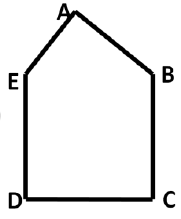B.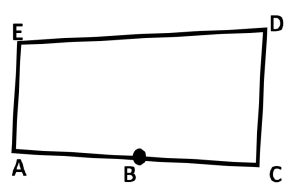C.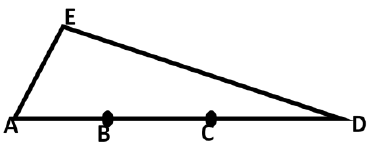D.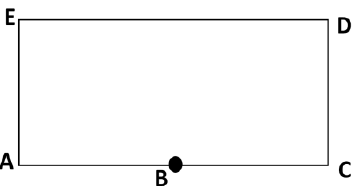NSEJS Maths Previous Year Questions Bank With Solution: Ques No 79

Number plate of a vehicle consists of 4 digits. The first digit is the square of second. The third digit is thrice the second and the fourth digit is twice the second. The sum of all 4 digits is thrice the first. The number is

[NSEJS 2014-15]

Options:

A. 1132

B. 4264

C. 9396

D. 1642

NSEJS Maths Previous Year Questions Bank With Solution: Ques No 85

Let m be the number of distinct (non-congruent) integer-sided triangles each with perimeter 15 and n be the number of distinct (non-congruent) integer sided triangles each with perimeter 16, then m – n equals

[NSEJS 2013-14]

Options:

A. -2

B. 0

C. 2

D. 4

NSEJS Maths Previous Year Questions Bank With Solution: Ques No 89

Sucharitha purchases x pencils at Rs. X each pens at Rs y each and z notebooks at Rs z each. She purchases all together 50 items and pays Rs. 1000. The cost of y pencils, z pens and x notebooks is

[NSEJS 2013-14]

Options:

A. Rs. 600

B. Rs. 750

C. Rs. 500

D. Rs. 350

NSEJS Maths Previous Year Questions Bank With Solution: Ques No 94

A certain principle becomes Rs 96800 in 2years if compounded annually at a certain rate of interest. The same principle becomes Rs. 97240 in two years if compounded half yearly at the same rate of interest. The rate of interest is

[NSEJS 2013-14]

Options:

A. 8%

B. 8(1/3)%

C. 10%

D. 12(1/2)%

NSEJS Maths Previous Year Questions Bank With Solution: Ques No 100

If the radius of the area of the base of a cone is doubled then the slant area becomes three times the original slant area. Suppose when the radius of the base of the cone is quadrupled (that is increased to 4 times), then the slant area becomes k times the original slant area. The integer closest to k is

[NSEJS 2013-14]

Options:

A. 6

B. 8

C. 10

D. 11

NSEJS Maths Previous Year Questions Bank With Solution: Ques No 112

In the adjoining figure, AQ = 2, QB = 4, BP = 3, PC = 5, CR = RA = 4. Find the area of the triangle PQR.[NSEJS 2012-13]

Options:

A. 4.8

B. 5.2

C. 5.8

D. 6.2

NSEJS Maths Previous Year Questions Bank With Solution: Ques No 119

The distance between two spots A and B on the same bank of the river is 75 km. Speed of the boat in still water is twice as much as that of the speed of the water current of the river. The boat travels in the river from A to B and returns back to spot in 16 hours. What is the speed of the boat still water?

[NSEJS 2012-13]

Options:

A. 12.5 km/h

B. 15 km/h

C. 16 km/h

D. 18 km/h

NSEJS Maths Previous Year Questions Bank With Solution: Ques No 123

An equilateral triangle has area A cm2. A regular hexagon of maximum area is cut off from the triangle. If the area of the hexagon is 320 cm2, then the area A is

[NSEJS 2012-13]

Options:

A. 640 cm2

B. 480 cm2

C. 600 cm2

D. 400 cm2

NSEJS Maths Previous Year Questions Bank With Solution: Ques No 129

A conical vessel of radius 6 cm and height 8 cm is completely filled with water. A metal sphere is now lowered into the water. The size of the sphere is such that when it touches the inner surface, it just gets immersed. The fraction of water that overflows from the conical vessel is

[NSEJS 2012-13]

Options:

A. 3/8

B. 5/8

C. 7/8

D. 5/16

NSEJS Maths Previous Year Questions Bank With Solution: Ques No 158

A point P is outside a circle at a distance of 13 cm from its centre. A secant from P cuts the circle in Q and R such that QR = 7 cm and the segment PQ of the secant exterior to the circle is 9 cm. Therefore, the radius of the circle is

[NSEJS 2010-11]

Options:

A. 3 cm

B. 4 cm

C. 5 cm

D. 6 cm

NSEJS Maths Previous Year Questions Bank With Solution: Ques No 168

In a rectangle ABCD, the lengths of sides AB, BC, CD and DA are (5x + 2y + 2) cm, (x + y + 4) cm, (2x + 5y – 7) cm and (3x + 2y – 11) cm respectively. Which of the following statements is/are true?

[NSEJS 2009-10]

Options:

A. One of the sides of the rectangle is 15 cm long

B. Each diagonal of the rectangle is 39 cm long

C. Perimeter of the rectangle is 102 cm

D. Area of the rectangle is 560 cm2